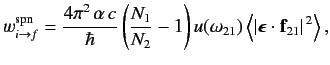$$\require{cancel}$$

# 8.11: Spontaneous Emission

So far, we have calculated the rates of radiation induced transitions between two atomic states. This process is known as absorption when the energy of the final state exceeds that of the initial state, and stimulated emission when the energy of the final state is less than that of the initial state. Now, in the absence of any external radiation, we would not expect an atom in a given state to spontaneously jump into a state with a higher energy. On the other hand, it should be possible for such an atom to spontaneously jump into an state with a lower energy via the emission of a photon whose energy is equal to the difference between the energies of the initial and final states. This process is known as spontaneous emission.

It is possible to derive the rate of spontaneous emission between two atomic states from a knowledge of the corresponding absorption and stimulated emission rates using a famous thermodynamic argument due to Einstein. Consider a very large ensemble of similar atoms placed inside a closed cavity whose walls (which are assumed to be perfect emitters and absorbers of radiation) are held at the constant temperature $$T$$. Let the system have attained thermal equilibrium. According to statistical thermodynamics, the cavity is filled with so-called black-body'' electromagnetic radiation whose energy spectrum is

$u(\omega) = \frac{\hbar}{\pi^2\,c^3}\,\frac{\omega^3}{\exp(\hbar\,\omega/k_B\,T)-1}, \label{900}$

where $$k_B$$ is the Boltzmann constant. This well-known result was first obtained by Max Planck in 1900.

Consider two atomic states, labeled $$2$$ and $$1$$ , with $$E_2> E_1$$ . One of the tenants of statistical thermodynamics is that in thermal equilibrium we have so-called detailed balance. This means that, irrespective of any other atomic states, the rate at which atoms in the ensemble leave state $$2$$ due to transitions to state $$1$$ is exactly balanced by the rate at which atoms enter state $$2$$ due to transitions from state $$1$$ . The former rate (i.e., number of transitions per unit time in the ensemble) is written

$$W_{2\rightarrow 1} = N_2\,(w_{2\rightarrow 1}^{\rm spn} + w_{2\rightarrow 1}^{\rm stm}),$$ \ref{901}

where $$w_{2\rightarrow 1}^{\rm spn}$$ and $$w_{2\rightarrow 1}^{\rm arm}$$ are the rates of spontaneous and stimulated emission, respectively, (for a single atom) between states $$2$$ and $$1$$ , and $$N_2$$ is the number of atoms in the ensemble in state $$2$$ . Likewise, the latter rate takes the form

$$W_{1\rightarrow 2} = N_1\,w^{\rm abs}_{1\rightarrow 2},$$ \ref{902}

where $$w^{\rm abs}_{1\rightarrow 2}$$ is the rate of absorption (for a single atom) between states $$1$$ and $$2$$ , and $$N_1$$ is the number of atoms in the ensemble in state $$1$$ . The above expressions describe how atoms in the ensemble make transitions from state $$2$$ to state $$1$$ due to a combination of spontaneous and stimulated emission, and make the opposite transition as a consequence of absorption. In thermal equilibrium, we have $$W_{2\rightarrow 1}=W_{1\rightarrow 2}$$ , which gives

$$w_{2\rightarrow 1}^{\rm spn} = \frac{N_1}{N_2}\,w^{\rm abs}_{1\rightarrow 2}- w_{2\rightarrow 1}^{\rm stm}.$$ \ref{903}

Equations \ref{891} and \ref{892} imply that\ref{904}

where $$\omega_{21} = (E_2 - E_1)/\hbar$$ , and the large angle brackets denote an average over all possible directions of the incident radiation (because, in equilibrium, the radiation inside the cavity is isotropic). In fact, it is easily demonstrated that

$$\left\langle \vert\mbox{\boldmath$$ \ref{905}

where $$f^{\,2}_{21}$$ stands for\ref{906}

Now, another famous result in statistical thermodynamics is that in thermal equilibrium the number of atoms in an ensemble occupying a state of energy $$E$$ is proportional to $$\exp(-E/k_B\,T)$$ . This implies that

$$\frac{N_1}{N_2} = \frac{\exp(-E_1/k_B\,T)}{\exp(-E_2/k_B\,T)} = \exp(\,\hbar\, \omega_{21}/k_B\,T).$$ \ref{907}

Thus, it follows from Equations \ref{900}, \ref{904}, \ref{905}, and \ref{907} that the rate of spontaneous emission between states $$2$$ and $$1$$ takes the form

$$w_{2\rightarrow 1}^{\rm spn} = \frac{\omega_{21}^{\,3}\,e^2\,f_{21}^{\,2}}{3\pi\,\epsilon_0\,\hbar\,c^3}.$$ \ref{908}

Note, that, although the above result has been derived for an atom in a radiation-filled cavity, it remains correct even in the absence of radiation.

Let us estimate the typical value of the spontaneous emission rate for a hydrogen atom. We expect the matrix element $$f_{21}$$ to be of order $$a_0$$ , where $$a_0$$ is the Bohr radius. We also expect $$\omega_{21}$$ to be of order $$\vert E_0\vert/\hbar$$ , where $$E_0$$ is the ground-state energy. It thus follows from Equation \ref{908} that

$$w_{2\rightarrow 1}^{\rm spn} \sim \alpha^3\,\omega_{21},$$ \ref{909}

where $$\alpha \simeq 1/137$$ is the fine structure constant. This is an important result, because our perturbation expansion is based on the assumption that the transition rate between different energy eigenstates is much slower than the frequency of phase oscillation of these states: i.e., that $$w_{2\rightarrow 1}^{\rm spn} \ll \omega_{21}$$ . This is indeed the case.

## Contributors

• Richard Fitzpatrick (Professor of Physics, The University of Texas at Austin)

$$\newcommand {\ltapp} {\stackrel {_{\normalsize<}}{_{\normalsize \sim}}}$$ $$\newcommand {\gtapp} {\stackrel {_{\normalsize>}}{_{\normalsize \sim}}}$$ $$\newcommand {\btau}{\mbox{\boldmath\tau}}$$ $$\newcommand {\bmu}{\mbox{\boldmath\mu}}$$ $$\newcommand {\bsigma}{\mbox{\boldmath\sigma}}$$ $$\newcommand {\bOmega}{\mbox{\boldmath\Omega}}$$ $$\newcommand {\bomega}{\mbox{\boldmath\omega}}$$ $$\newcommand {\bepsilon}{\mbox{\boldmath\epsilon}}$$